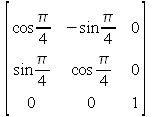Home > Resources > MBDyn Tutorial > 5. Free-Falling Body (3) - Defining rigid body: structural node and body
MBDyn Tutorial

## 5. Free-Falling Body (3) - Defining rigid body: structural node and body

We continue to analyze the input file for Problem 1, "free_falling_body.mbd." In this chapter, the contents of the nodes block and the elements block are treated. Here, a "structural node" and a "body" element, both of which are the most fundamental entities in constructing a simulation model, appear.

### Structural node

A node is an owner of kinematic degrees of freedom and is a fundamental entity in constructing a simulation model. There are several types of nodes:

• structural
• electric
• abstract
• parameter
• hydraulic

Among them, the one that can assume the six degrees of freedom for a rigid body is a structural node. Since in Problem 1 we have one rigid body, we need to define one structural node in our virtual space. If there are two rigid bodies, then we need two structural nodes. In general, if we are to simulate the motions of N rigid bodies, we need N structural nodes. There are four types of structural nodes: static, dynamic, modal, and dummy. To have a structural node assume the six degrees of freedom for a rigid body, we need the dynamic type.

The basic syntax for the statement that defines a dynamic structural node is as follows.

``` structural: <node label>, dynamic, <position>, <orientation>,
<velocity>, <angular velocity> ;
```

In the nodes block of the input file for Problem 1, one structural node is defined:

```   structural: 1, dynamic, null, eye, 0., 3., 0., null;
```

If we compare this statement with the above basic syntax, we have

• <node label> = 1
• <position> = null
• <orientation> = eye
• <velocity> = 0., 3., 0.
• <angular velocity> = null

Thus, a dynamic structural node with the label "1" is defined with the initial position "null" (= [0., 0., 0.]), the initial orientation "eye" (= identity matrix), the initial velocity "0., 3., 0." (3.0 in Y direction), and the initial angular velocity "null" (= [0., 0., 0.]). Note that the initial velocity of the rigid body is reflected in the initial velocity of the structural node.

In general, <node label> is an integer, <position>, <velocity>, and <angular velocity> are three-dimensional vectors (3x1 vectors), and <orientation> is a 3x3 orientation matrix. A null vector can be indicated by a keyword "null," and an identity matrix can be indicated by a keyword "eye."

While it is obvious how a three-dimensional vector is defined (by lining up three numbers), it is not obvious how a general 3x3 orientation matrix other than eye is defined. There are actually several ways. The most straightforward method is to use a keyword "matr" followed by nine matrix elements row-wise. For example, an orientation matrix representing a 45 degree-rotation about Z-axiscan be defined by

```   matr, cos(pi/4.), -sin(pi/4.), 0., sin(pi/4.), cos(pi/4.), 0., 0., 0., 1.,
```

In fact, eye is equivalent to

```   matr, 1., 0., 0., 0., 1., 0., 0., 0., 1.,
```

Other methods to define an orientation matrix and a general matrix are discussed in Chapter 13.

### Body

A structural node provides the degrees of freedom for a rigid body but it does not define a rigid body. To define a rigid body, information of mass, center of mass, and inertia tensor is required. It is an element called body that carries that information and it is defined in the elements block.

The basic syntax for the statement that defines a body is as follows.

``` body: <label>, <node label>, <mass>, <relative center of mass>,
<inertia matrix> ;
```

In the elements block of the input file for Problem 1, one body is defined:

```   body: 1, 1, 1., null, eye;
```

If we compare this statement with the above basic syntax, we have

• <label> = 1
• <node label> = 1
• <mass> = 1.
• <relative center of mass> = null
• <inertia matrix> = eye

Thus, a body with the label "1" is defined and attached to the node "1." The body has mass of "1.", the center of mass at "null" (= [0., 0., 0.]) relative to the node, and the inertia matrix "eye" (identity matrix).

In general, <label> is an integer, <node label> is the label of an existing node, <mass> is a real number, <relative center of mass> is a three-dimensional vector (3x1 vector), and <inertia matrix> is a 3x3 matrix. A null vector can be indicated by a keyword "null," and an identity matrix can be indicated by a keyword "eye."

### Gravity

The last statement is the one that defines the gravity:

```   gravity: 0., 0., -1., const, 9.81;
```

As can be easily guessed, "0., 0., -1." defines the direction of the gravity (-Z direction), and "const, 9.81" defines the magnitude of the gravity acceleration (constant value). To be precise, the argument for the gravity card is an object called "template drive caller" (See Chapter 19) and the above statement is an abbreviation of the statement below.

```   gravity: single, 0., 0., -1., const, 9.81;
```